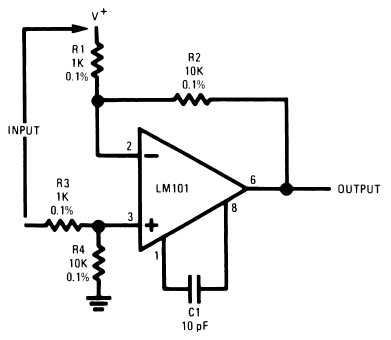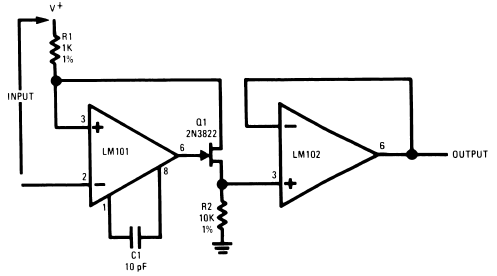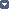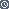## Level Shifting Amplifier

### By Robert J. Widlar

Level-Shifting Amplifiers: Standard Differential Amplifier and Level-Isolation Amplifier.

Frequently, in the design of linear equipment, it is necessary to take a voltage which is referred to some dc level and produce an amplified output which is referred to ground. The most straight-forward way of doing this is to use a differential amplifier similar to that shown in Figure 1. This circuit, however, has the disadvantages that the signal source is loaded by current from the input divider, R3 and R4, and that the feedback resistors must be very well matched to prevent erroneous outputs from the common mode input signal.Figure 1. Standard Differential AmplifierFigure 2. Level-Isolation Amplifier

A circuit which does not have these problems is shown in Figure 2. Here, an FET transistor on the output of the operational amplifier produces a voltage drop across the feedback resistor, R1, which is equal to the input voltage. The voltage across R2 will then be equal to the input voltage multiplied by the ratio, R2/R1; and the common mode rejection will be as good as the basic rejection of the amplifier, independent of the resistor tolerances. This voltage is buffered by an LM102 voltage follower to give a low impedance output.

An advantage of the LM101 in this circuit is that it will work with input voltages up to its positive supply voltages as long as the supplies are less than ±15V.627308 December 2007